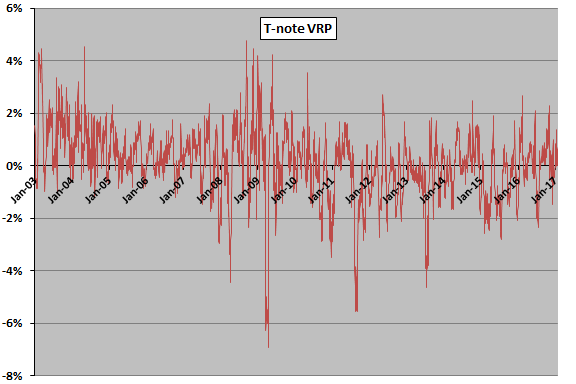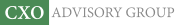Objective research to aid investing decisions

#### Value Investing Strategy (Strategy Overview)

Allocations for September 2023 (Final)
Cash TLT LQD SPY

#### Momentum Investing Strategy (Strategy Overview)

Allocations for September 2023 (Final)
1st ETF 2nd ETF 3rd ETF

# Simple, Practical Test of VRP as IEF Return Predictor

| | Posted in: Bonds, Volatility Effects

“Equity Market and Treasuries Variance Risk Premiums as Return Predictors” reports a finding, among others, that the variance risk premium for 10-year U.S. Treasury notes (T-note) predicts near-term returns for those notes (as manifested via futures). However, the methods used to calculate the variance risk premium are complex. Is there a simple way to exploit the predictive power found? To investigate, we test whether a simple measure of the volatility risk premium (VRP) for T-notes predicts returns for the iShares 7-10 Year Treasury Bond (IEF) exchange-traded fund. Specifically we:

• Calculate daily realized volatility of IEF as the standard deviation of daily total returns over the past 21 trading days, multiplied by the square root of 252 to annualize.
• Use daily closes of CBOE/CBOT 10-year U.S. Treasury Note Volatility Index (TYVIX) as annualized implied volatility.
• Calculate the daily T-note VRP as TYVIX minus IEF realized volatility.

VRP here differs from that in the referenced research in three ways: (1) it is a volatility premium rather than a variance premium based on standard deviation rather than the square of standard deviation; (2) it is implied volatility minus expected realized volatility, rather than the reverse, and so should be mostly positive; and, (3) estimation of expected realized volatility is much simpler. When TYVIX has daily closes on non-market days, we ignore those closes. When TYVIX does not have daily closes on market days, we reuse the most recent value of TYVIX. These exceptions are rare. Using daily IEF dividend-adjusted prices since December 2002 and daily closes of TYVIX since January 2003 (earliest available), both through January 2017, we find that:

The following chart tracks daily T-note VRP over the available sample period. Notable findings are:

• The average daily premium is just 0.12%.
• The daily premium is very volatile, with standard deviation 1.33% (about 11 times the average).
• While daily readings are mostly positive, the premium is non-positive on 42% of days.
• It appears there may be two regimes, with break point about the end of 2010. Since 2010, the premium is non-positive on 56% of days.

How does daily T-note VRP relate to future IEF returns?The next chart summarizes correlations between daily T-note VRP and daily IEF return for various lead-lag relationships, ranging from IEF return leads T-note VRP by 21 trading days (-21) to T-note VRP leads IEF return by 21 trading days (21). While small correlations may be meaningful for daily data, in this case T-note VRP appears to have no relationships with IEF returns over the next 21 trading days. The average correlation for T-note VRP leads IEF returns by 1 through 21 days is 0.01.

Because T-note VRP is so noisy, we also relate its 21-day simple moving average calculated at the end of each month to next-month IEF return. The correlation is only 0.01, and a simple trading rule that avoids IEF when the prior-month T-note VRP is negative loses average return faster than it loses volatility. In other words, it is unattractive even with simple risk adjustment.In summary, evidence from simple tests with a very simple estimation of T-note VRP does not support belief that it usefully predicts near-term returns for a T-note proxy.

Cautions regarding findings include:

• The very simple approach to estimating future T-note realized return volatility may not reflect investor view of likely actual risk.
• The available sample is short in terms of number of interest rate, inflation and volatility regimes.